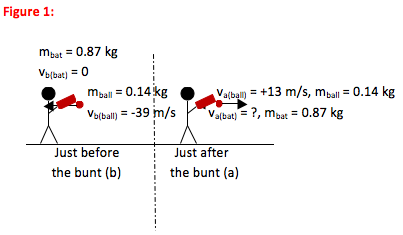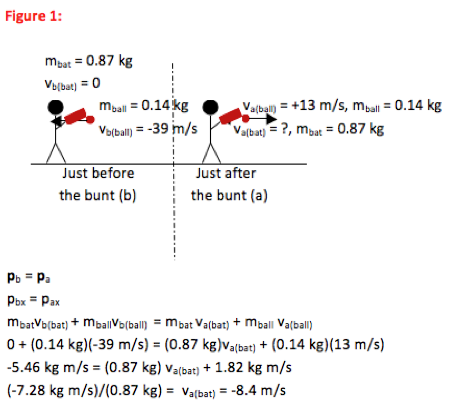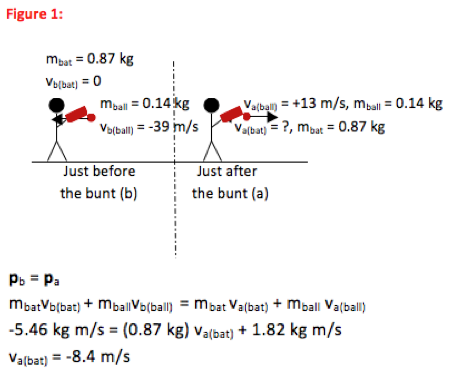# University of Wisconsin Green Bay

A 0.14 kg baseball is pitched with a speed of 39 m/s. If the batter bunts the ball so that it returns toward the pitcher with a speed of 13 m/s, what is the velocity of the bat immediately after the bunt? Treat this as a one-dimensional problem with the bat allowed to move freely during impact. The mass of the bat is 0.87 kg.

• In this problem, you are asked to find the velocity of a bat immediately after impact (collision) with a ball. Any time you are asked to find speed or velocity of an object just before or just after a collision or separation, you should check to see if you can use Conservation of Momentum to solve the problem.

In this case, the force of impact between the bat and the ball is much larger than any other forces that act on that system. (The problem hinted at this when it told you to treat the bat as moving freely.) You can, indeed, use Conservation of Momentum.

•For Conservation of Momentum problems, always draw a picture of the system immediately before the collision or separation and another picture immediately after. Because momentum depends on mass and velocity, label all mass and velocity information

•Pb = Pa

Any time you understand the motion of a system for which Fextermal Δt≈0, you begin with the Conservation of Momentum equation.

•Immediately after the ball is bunted, the bat moves backwards with a velocity of 8.4 m/s towards the batter. No further mathematical solution is needed for this problem.

•In this problem, you are asked to find velocity of a bat immediately after it was hit with a ball. This is a collision problem and so you should first consider solving it using Conservation of Momentum. Because the force of impact is so much larger than other forces acting on the bat and ball, you are able to ignore any effect of external forces during the time of collision and so conditions to use Conservation of Momentum are, indeed, met.

In this case, over half of the initial kinetic energy of the baseball is lost from the system as heat and sound. (You can find this by calculating the kinetic energies of the bat and ball before and after collision.) So if you were unconvinced earlier that the problem can’t be solved using energy, you can see that now.

Physically, note that the change in momentum of the ball is (0.14 kg)(13 m/s –(-39 m/s)) not (0.14 kg)(39 m/s). In other words, there is a greater change in momentum to reverse direction of an object than to stop it. This should make sense—it hurts more to hit a ball hard enough to send it away from you than it does to stop the ball.